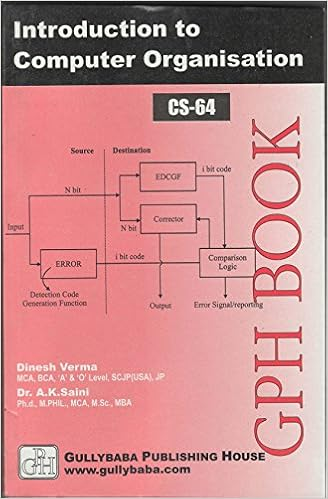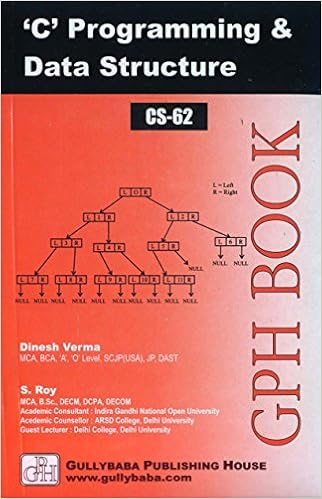IGNOU BCA MCA.comQuestion Papers of IGNOU BCA CS62

 CS62: 'C' programming and Data StructureYear: 2002 TEE: December Time 2 Full Marks 60 Note: There are 6 questions in this paper. Question 1 is compulsory. Answer any three questions from the rest. All algorithms should be written nearer to ?c? language.Q.2(b): Device a scheme using a stack to convert an infix expression to a postfix expression. Q.3(a): Write the postfix form of the following expression: (i) a + b x (c ÷ d) (ii) (a + b x c) ÷ d: Q.3(b): Write an algorithm for multiplication of two matrices.: Q.4(a): Devise an algorithm to count the number of connected components in a directed graph.: home || << Previous || Next >>